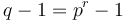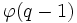# Multiplicative group of a finite field is cyclic

## Statement

Suppose$F$ is a finite field. Let$F^*$ denote the multiplicative group of$F$. Then,$F^*$ is a cyclic group.

## Related facts

### Consequences

• Note in particular that any finite field has order a prime power, say$q = p^r$ for a prime number$p$ and positive integer$r$. The multiplicative group is therefore a cyclic group of order$q - 1 = p^r - 1$.
• Since the multiplicative group is cyclic, there are$\varphi(q - 1)$ many choices for the generator of the group. Here,$\varphi$ is the Euler totient function.
• Multiplicative group of a finite prime field is cyclic (see also classification of natural numbers for which the multiplicative group is cyclic)

### Other related facts

• For$r > 1$, any generator of the multiplicative group is also a primitive element for the field of$q$ elements as an extension of its prime subfield (of$p$ elements). (A primitive element for a field extension is an element that, when adjoined to the smaller field, generates the larger field). However, not every primitive element is a generator of the multiplicative group. In fact, the number of generators of the multiplicative group could be substantially smaller than the number of primitive elements. For instance, consider the case$p = 5, r = 2$. The multiplicative group has$\varphi(24) = 8$ generators, whereas the field has$p^2 - p = 25 - 5 = 20$ primitive elements.
• Every finite division ring is a field

## Facts used

1. Multiplicative group of a field implies every finite subgroup is cyclic

## Proof

The statement follows directly from fact (1).